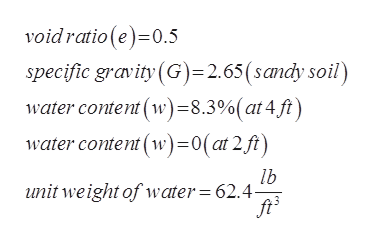# An excavation was made in sandy soil and soilsamples were collected at depths of 2ft 4ft,and 6 ft. Ground water was encountered at depth of 5 ft. Soil sample at 2ftwas dry. Soilsample at 4ft was tested in the lab and moisture content of 8.3% was determined. Assuming void ratio of 0.5 determine:a)Unit weight (lb/ft3) of soil at 2ft(110.24pcf)b)Unit weight (lb/ft3) of soil at 4ft(119.39pcf)c)Moisture content (%) of soil at 6ft(18.87%)

Question
3 views
An excavation was made in sandy soil and soil
samples were collected at depths of 2ft 4ft,
and 6 ft. Ground water was encountered at depth of 5 ft. Soil sample at 2ft
was dry. Soilsample at 4ft was tested in the lab and moisture content of 8.3% was determined. Assuming void ratio of 0.5 determine:
a)Unit weight (lb/ft3) of soil at 2ft(110.24pcf)
b)Unit weight (lb/ft3) of soil at 4ft(119.39pcf)
c)Moisture content (%) of soil at 6ft(18.87%)
check_circle

Step 1

We have been given the following information:help_outlineImage Transcriptionclosevoidratio (e) 0.5 specific gravity (G)=2.65(sandy soil) water content (w)=8.3%(at4 ft) water content w)=0(at2 ft) lb unit weight of water 62.4- fullscreen
Step 2

With given data we can draw the following diagram:

Step 3

a) Bulk unit weight at 2ft: ...

### Want to see the full answer?

See Solution

#### Want to see this answer and more?

Solutions are written by subject experts who are available 24/7. Questions are typically answered within 1 hour.*

See Solution
*Response times may vary by subject and question.
Tagged in

### Civil Engineering